# Math in Focus Grade 4 Chapter 7 Practice 2 Answer Key Understanding Hundredths

Practice the problems of Math in Focus Grade 4 Workbook Answer Key Chapter 7 Practice 2 Understanding Hundredths to score better marks in the exam.

## Math in Focus Grade 4 Chapter 7 Practice 2 Answer Key Understanding Hundredths

Shade the squares to represent each decimal.
Each large square represents 1 whole.

Example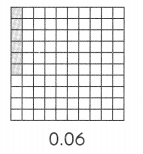Question 1.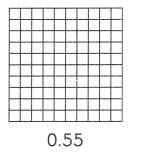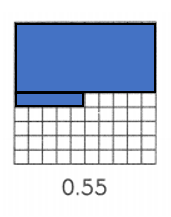Explanation:
0.55 = 0.50 + 0.05.

Question 2.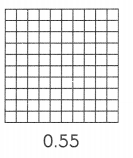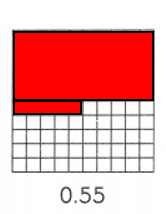Explanation:
0.55 = 0.50 + 0.05.

Question 3.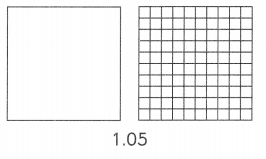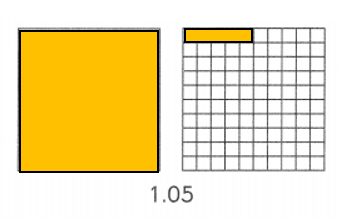Explanation:
1.05 = 1.00 + 0.05.

Write a decimal for each place-value chart.
Question 4.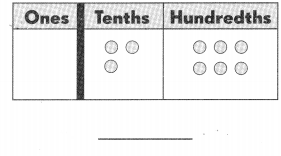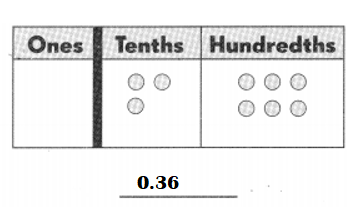Explanation:
0 ones 3 tenths 6 hundredths = 0.36.

Question 5.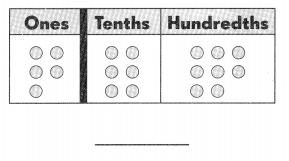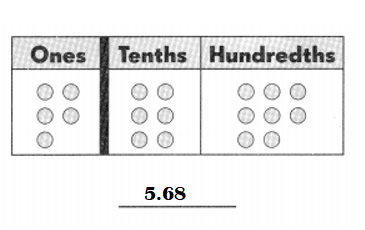Explanation:
5 ones 6 tenths 8 hundredths = 5.68.

Question 6.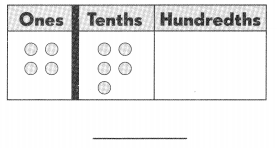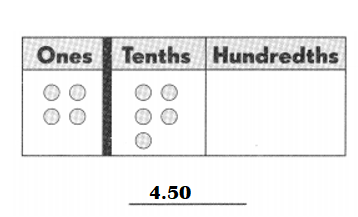Explanation:
4 ones 5 tenths 0 hundredths = 4.50.

Question 7.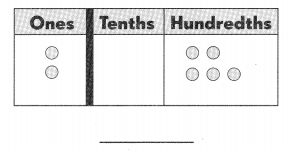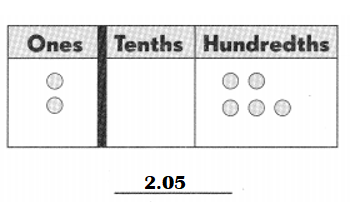Explanation:
2 ones 0 tenths 5 hundredths = 2.05.

Write the correct decimal in each box.

Question 8.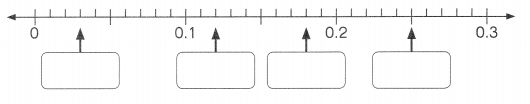Answer: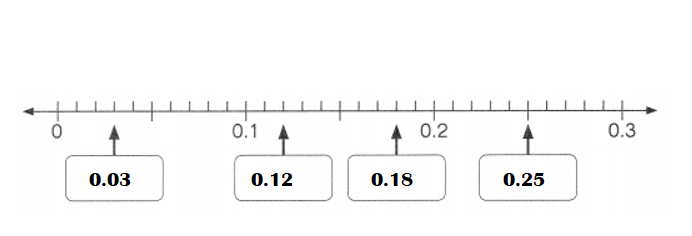Explanation:
0 + 0.03 = 0.03.
0.1 + 0.02 = 0.12.
0.12 + 0.06 = 0.18.
0.2 + 0.05 = 0.25.

Mark ✗ t0 show where each decimal is located on the number line. Label its value.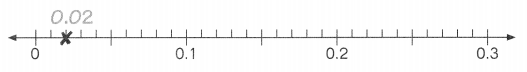Question 9.
0.14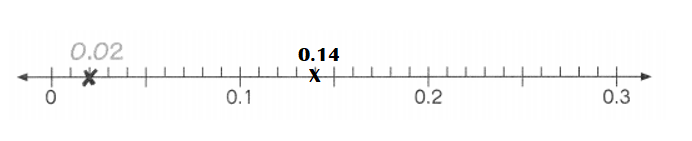Explanation:
0.1 + 0.04 = 0.14.

Question 10.
0.22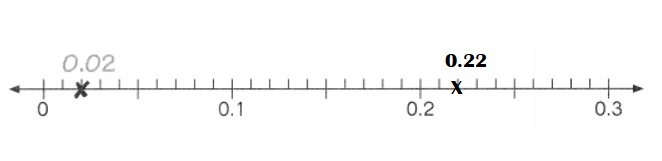Explanation:
0.20 + 0.02 = 0.22.

Question 11.
0.27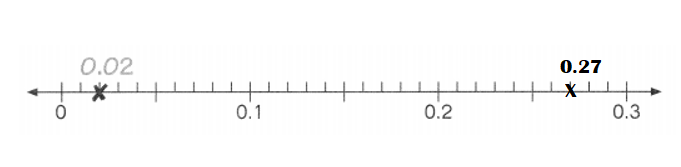Explanation:
0.20 + 0.07 = 0.27.

Write each of these as a decimal.
Question 12.
9 hundredths = ___________
9 hundredths = 0.09.

Explanation:
9 hundredths = 9 ÷ 100 =  0.09.

Question 13.
23 hundredths = __________
23 hundredths = 0.23.

Explanation:
23 hundredths = 23 ÷ 100 = 0.23.

Question 14.
6 tenths 1 hundredth = ___________
6 tenths 1 hundredth = 0.61.

Explanation:
6 tenths 1 hundredth = 0.61.

Question 15.
7 ones and 90 hundredths = __________
7 ones and 90 hundredths = 7.090.

Explanation:
7 ones and 90 hundredths = 7.090.

Write each fraction as a decimal.
Question 16.
$$\frac{5}{100}$$ = _______
$$\frac{5}{100}$$ = 0.05.

Explanation:
$$\frac{5}{100}$$ = 0.05.

Question 17.
$$\frac{19}{100}$$ = _______
$$\frac{19}{100}$$ = 0.19.

Explanation:
$$\frac{19}{100}$$ = 0.19.

Question 18.
$$\frac{83}{100}$$ = _______
$$\frac{83}{100}$$ = 0.83.

Explanation:
$$\frac{83}{100}$$ = 0.83.

Question 19.
$$\frac{70}{100}$$ = _______
$$\frac{70}{100}$$ = 0.70.

Explanation:
$$\frac{70}{100}$$ = 0.70.

Write each fraction or mixed number as a decimal.
Question 20.
3$$\frac{17}{100}$$ = _______
3$$\frac{17}{100}$$ = 3.17.

Explanation:
3$$\frac{17}{100}$$ = (300 + 17) ÷ 100
= $$\frac{317}{100}$$
= 3.17.

Question 21.
18$$\frac{9}{100}$$ = _______
18$$\frac{9}{100}$$ = 18.09.

Explanation:
18$$\frac{9}{100}$$ = (1800 + 9) ÷ 100
= $$\frac{1809}{100}$$
= 18.09

Write each fraction or mixed number as a decimal.
Question 22.
$$\frac{233}{100}$$ = _______
$$\frac{233}{100}$$ = 2.33.

Explanation:
$$\frac{233}{100}$$ = 2.33.

Question 23.
$$\frac{104}{100}$$ = _______
$$\frac{104}{100}$$ =1.04.

Explanation:
$$\frac{104}{100}$$ = 1.04.

Write each decimal in hundredths.
Question 24.
0.07 = __________ hundredths
0.07 = 7 hundredths.

Explanation:
0.07 = 7 ÷ 100 = 7 hundredths.

Question 25.
2.31 = __________ hundredths
2.31 = 231 hundredths.

Explanation:
2.31 = 231 ÷ 100 = 231 hundredths.

Question 26.
1.83 = __________ hundredths
1.83 = 183 hundredths.

Explanation:
1.83 = 183 ÷ 100 = 183 hundredths.

Question 27.
5.09 = __________ hundredths
5.09 = 509 hundredths.

Explanation:
5.09 = 509 ÷ 100 = 509 hundredths.

Fill in the blanks.
Question 28.
0.38 = __________ tenths 8 hundredths
0.38 = 3 tenths 8 hundredths.

Explanation:
0.38 = 3 tenths 8 hundredths.

Question 29.
2.71 = 2 ones and 7 tenths __________ hundredth
2.71 = 2 ones and 7 tenths 1 hundredth.

Explanation:
2.71 = 271 ÷ 100 = 2 ones and 7 tenths 1 hundredth.

Question 30.
5.09 = 5 ones and __________ hundredths
5.09 = 5 ones and 9 hundredths.

Explanation:
5.09 = 509 ÷ 100 = 5 ones and 9 hundredths.

Question 31.
8.86 = 8 ones and 8 tenths ___________ hundredths
8.86 = 8 ones and 8 tenths 6 hundredths.

Explanation:
8.86 = 886 ÷ 100 = 8 ones and 8 tenths 6 hundredths.

You can write 6.13 in expanded form as 6 + $$\frac{1}{10}$$ + $$\frac{3}{100}$$. Complete in the same way.
Question 32.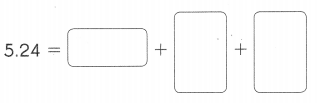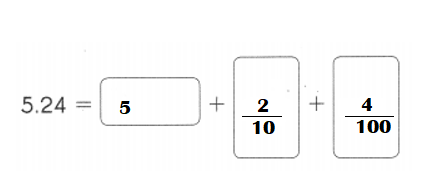Explanation:
5.24 = 5 + $$\frac{2}{10}$$ + $$\frac{4}{100}$$
= 5 + 0.20 + 0.04.

Question 33.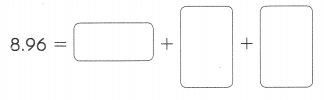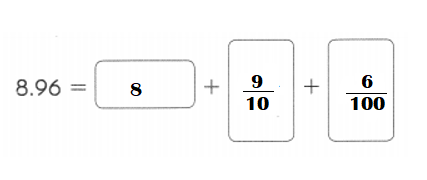Explanation:
8.96 = 8 + $$\frac{9}{10}$$ + $$\frac{6}{100}$$
= 8 + 0.90 + 0.06.

You can write 7.45 in expanded form as 7 +0.4 + 0.05. Complete in the same way.
Question 34.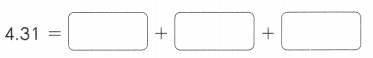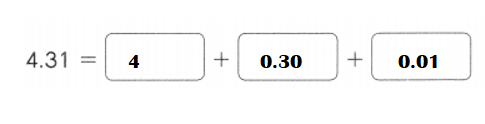Explanation:
4.31  = 4 + 0.30 + 0.01.

Question 35.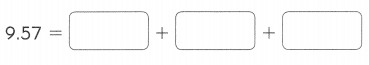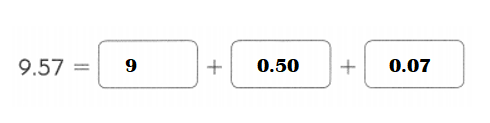Explanation:
9.57 = 9 + 0.50 + 0.01

Fill in the blanks.
Question 36.
In 0.38, the digit 8 is in the ____ place.
In 0.38, the digit 8 is in the hundredths place.

Explanation:
In 0.38,
=> 0 ones 3 tenths 8 hundredths.
The digit 8 is in the hundredths place.

Question 37.
In 1 2.67, the digit in the tenths place is ___.
In 1 2.67, the digit in the tenths place is 6.

Explanation:
In 1 2.67,
=> 1 tens 2 ones 6 tenths 7 hundredths.
The digit in the tenths place is 6.

Question 38.
In 3.45, the value of the digit 5 is ____.
In 3.45, the value of the digit 5 is hundredths(0.05).

Explanation:
In 3.45,
=> 3 ones 4 tenths 5 hundredths.
The value of the digit 5 is hundredths(0.05).

Question 39.
In 5.02, the value of the digit 2 is ___________ hundredths.
In 5.02, the value of the digit 2 is 0.02 hundredths.

Explanation:
In 5.02,
=> 5 ones 0 tenths 2 hundredths.
The value of the digit 2 is 0.02 hundredths.

Write each amount in decimal form.
Question 40.
75 cents = $_______ Answer: 75 cents =$ 0.75.

Explanation:
75 cents = $?? Conversion: 1 dollar = 100 cents. => 75 cents = 75 ÷100 =$0.75.

Question 41.
40 cents = $_______ Answer: 40 cents =$0.40.

Explanation:
40 cents = $?? Conversion: 1 dollar = 100 cents. => 40 cents = 40 ÷100 =$0.40.

Question 42.
5 cents = $____ Answer: 5 cents =$0.05.

Explanation:
5 cents = $?? Conversion: 1 dollar = 100 cents. => 5 cents = 5 ÷100 =$0.05.

Question 43.
130 cents = $____ Answer: 130 cents =$ 1.30.

Explanation:
130 cents = $?? Conversion: 1 dollar = 100 cents. => 130 cents = 130 ÷100 =$ 1.30.

Question 44.
10 dollars and 25 cents = $____ Answer: 10 dollars and 25 cents =$ 10.25.

Explanation:
10 dollars and 25 cents = $?? Conversion: 1 dollar = 100 cents. => 10 dollars and 25 cents =$10 +$(25 ÷100) =$10 + $0.25 =$10.25.

Question 45.
28 dollars = $____ Answer: 28 dollars =$28.00.

Explanation:
28 dollars = $??. Conversion: 1 dollar = 100 cents. => 28 dollars =$28.00.

Question 46.
1 dollar and 9 cents = $____ Answer: 1 dollar and 9 cents =$1.09.

Explanation:
1 dollar and 9 cents = $?? Conversion: 1 dollar = 100 cents. => 1 dollar and 9 cents =$1 + $(9 ÷ 100) =>$1 + $0.09 =>$1.09.